Binary Addition, Binary Subtraction – One’s Complement, Two’s Complement Method, Binary Multiplication, Binary Division : Pharmaguideline -->
 Editable Pharmaceutical Documents in MS-Word Format

# Binary Addition, Binary Subtraction – One’s Complement, Two’s Complement Method, Binary Multiplication, Binary Division

'Binary Operation' refers to the mathematical operation of using two operands to perform one mathematical operattion.

Binary operations include binary addition. The term 'Binary Operation' refers to the mathematical operation of using two operands to perform one mathematical operation. In mathematics, addition, subtraction, multiplication, and division are the four arithmetic operations. Based on the base-2 system, binary addition also works the same way as decimal addition, except it is a base-2 system. There are only two digits in the binary system: 1 and 0. Binary numbers are used in almost all aspects of computing. Certain processes are turned off or on using binary code, which uses 1's and 0's. With the base 2 system, it is very familiar to perform addition operations in the decimal system. In order to be able to add binary numbers, it is imperative that we understand the binary number system. The binary operation is always performed by representing each bit as a voltage signal in modern digital computers and electronic circuits. An ON bit is represented by bit 1, and an OFF bit by bit 0.

0 + 1 = 1

1 + 1 = 10

1 + 0 = 1

0 + 0 = 0

The following table shows how to add two binary numbers 0 and 1: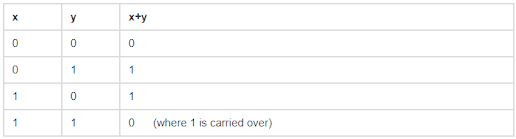The binary numbers x and y are shown in the above table. In other words, when we input 0 for x and 0 for y, we will get 0 as the output. It follows that x+y = 1 when x is 0 or 1 and y is 0 or 1. The addition of both x and y will equal 0, but the carryover number will equal 1, meaning one + one equals 10 in binary addition, where one is carried forward.

## Binary Subtraction

Subtracting binary numbers is known as binary subtraction. Binary numbers contain only 0's and 1's. Subtraction is the same operation we do with numbers and binary is no different. In this case, we can sometimes subtract 0 from 1 since only 0 and 1 are concerned. Borrowing can be used instead of arithmetic subtraction in such cases. Base-2 is used to express a binary number. An example would be: 1012.

Determinants can be represented as binary numbers, and base-10 numbers can be represented as binary numbers. Computers only understand binary numbers, 0 and 1, therefore their data is represented using binary numbers. This example explains how binary numbers can be subtracted.

### Case 1 - Subtraction of binary numbers without borrowing

#### Step 1

In the figure below, the numbers have been arranged.#### Step 2

Subtract the numbers according to binary subtraction rules. The subtraction of 1 from 0 does not occur in this subtraction. This results in a difference of 10112.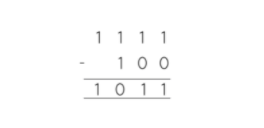#### Step 3

The decimal equivalent of 10112 is 11. They are different in this regard.

### Case 2 - Borrowing and subtracting in binary

#### Step 1

Put the numbers in the order shown below.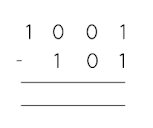#### Step 2

To subtract the numbers, you must follow binary subtraction rules. Starting from the right, let's subtract the numbers and then go to the next higher order digit. We start by subtracting (1-1). Therefore, we have a zero. In the same way, we add (0 - 0), resulting in 0. To do this, we have to subtract (0 - 1), which is why we borrow a 1 from the next higher order digit. As a result, we get 1.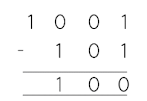### One's Complement Method

Digital electronics use binary number systems for representing numbers. Binary complements are used to represent negative decimal numbers. A binary number can have different types of complements, but the 1's and 2's complements are the most common. By simply inverting the binary number, we can find the binary number's 1's complement. Adding 1 to the least significant bit and changing each bit from 0 to 1 we can find the 2's complement of any binary number. In binary numbers (1011001), 2's complement is (0100110 )+ 1=0100111. The NOT gate can also be used in implementing the logic circuit to find the binary number's 1's complement. Each binary bit can be implemented by NOT gate. Hence, if we want a logic circuit with 5 bits 1's complement, 5 NOT gates will be necessary.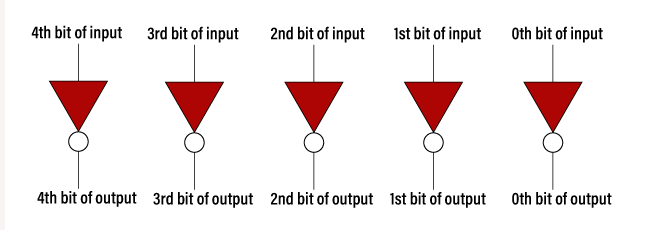Example – 11010.1101

You can get the 1's complement of the given number by replacing all 0's with 1's and all 1's with 0's. 11010.1101 would be 00101.0010 if the 1's complement was added.

Example – 100110.1001

The 1's complement of a number can be determined by treating all 0's as 1's and all 1's as 0. It is therefore 011001.0110, the 1's complement of 100110.1001.

Table -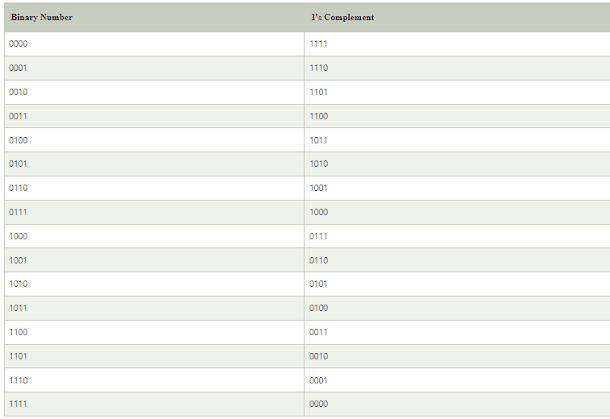1's complement is used to represent signed binary numbers. A signed binary number is typically represented by 1's complement. Arithmetic operations, including additions and subtractions, can be performed with it as well. Positive and negative numbers can be represented by a signed binary number. The representation of positive numbers is straightforward. The 1's complement method is used for representing negative numbers. Negative numbers can be represented by representing them with a positive sign, and then finding their 1's complement.

### Two’s Complement Method

In digital systems, Binary Number Systems are among the most popular methods for representing numbers. 0 (off) and 1 (on) are the only possible binary symbols or digit values in the Binary System. A device that can operate in only two states, Binary numbers usually have two complements: the complement of 1 and the complement of 2. The 1's complement is obtained by inverting the given number. The binary number 110010 has a complement of 001101. The 2's complement of a binary number is determined by adding 1 to the least significant bit (LSB) and 1 to the complement of the 1's.

Binary numbers can be converted into 2's complement using a simple algorithm. To invert a binary number, add 1 to its least significant bit (LSB), and invert the result. In the following table, we discuss how to implement 4-bit 2's complementation number.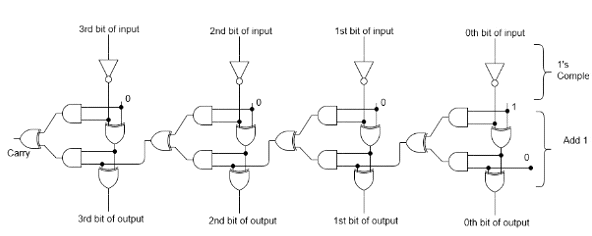### Binary Multiplication

Multiplication of binary numbers is known as binary multiplication. Binary numbers can be multiplied just like decimal numbers in arithmetic multiplication. 0s and 1s are the only factors that differ when it comes to binary multiplication, whereas digits from 0 to 9 are involved in decimal multiplication.

A binary number multiplied by a decimal number gives the same result. There are multipliers and multiplicands involved. We get a product when we multiply them. Multiplication of binary digits only involves 0s and 1s because binary digits are only comprised of binary digits. There are a lot of binary multiplication rules.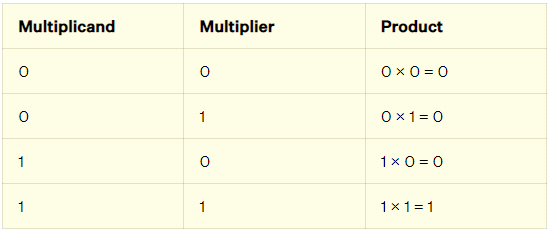Multiplying binary numbers is similar to multiplying decimal numbers because binary numbers are only made up of two digits, 0 and 1. Below is how to multiply binary numbers. Similarly, binary numbers denoted by a decimal point follow the same rules. For instance, multiplying a binary number with a decimal point (1001)2 and (11101)2. (11101)2 is equal to 29 decimal places, while (1001)2 is equal to nine decimal places.

## Binary Division

As part of binary arithmetic, binary division is an important operation. With a binary number system, you can represent a number with 0 and 1 and use a base 2 representation. A binary number is one with a base of two and only two digits, 0 and 1. The prefix 'bi' means 'two' in this context. Most computers use binary number systems. There are just two symbols used in binary number systems, 0 and 1, by all computers. The rapid development of technologies across the globe makes it extremely important for one to be aware of the binary number system.

Just like other binary operations, binary division requires a binary number. In binary division, the rules are similar to those used in decimal division, although the digits '0' and '1' are used differently. There are two binary operations associated with binary division: binary multiplication and binary subtraction. Since binary division uses only '0' and '1', binary division is easier than decimal division. The binary multiplication and binary subtraction operations are also used when performing binary division.

In addition to addition, subtraction, multiplication, and division, binary numbers can be used for all arithmetic operations. In this section, four different types of arithmetic operations are performed: binary addition, binary multiplication, and binary division. Dividing two binary numbers is as simple as following a few rules. Binary division requires following four rules. In binary division, division by 0 is also meaningless, just like it is in decimal division (or any other number system). There are three rules for binary division: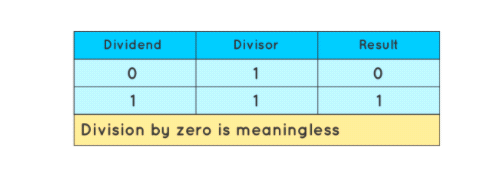As binary numbers only have two digits 0 and 1, the four rules given above cover all possible conditions for splitting binary numbers. When dividing a binary number, long division is one of the simplest and most efficient methods. Here are the steps to follow when dividing binary numbers:
• Step 1 - Dividends are multiplied by divisors. After dividing by the divisor, place 0 as the quotient and bring the second bit down. The subtraction is the result of the smaller divisor. The remainder is the subtrahend minus the minimum.
• Step 2 - Step 1 should be repeated after you have brought down the next bit of the dividend portion.
• Step 3 - Divide the dividend until there is no remainder or until you divide the whole dividend.
When it comes to binary division, you should remember the following points.
• The binary arithmetic operations of multiplication and subtraction include addition as well as division.
• As with regular division, we follow a similar process when dividing binary numbers. In this case, instead of deciding whether it will be a 1 or a 0, we only have to decide if it's a 1.
• One of the easiest and most efficient ways of solving binary division problems is to use the long division method.
• It is commonly found in computer technology to use binary numbers.
Get subject wise printable pdf documentsAnkur Choudhary is India's first professional pharmaceutical blogger, author and founder of pharmaguideline.com, a widely-read pharmaceutical blog since 2008. Sign-up for the free email updates for your daily dose of pharmaceutical tips.

## Popular Categories

QA SOPs QC SOPs Micro SOPs HVAC Production SOPs Stores SOPs Checklists Maintenance SOPs HPLC Sterile GLP Validation Protocols Water System GDP Regulatory Maintenance Calibration Warning Letters Education B.Pharmacy
Online Courses

## DOCUMENTS

PHARMACEUTICAL DOCUMENTS

Editable Pharmaceutical Documents in MS-Word Format. Ready to use SOPs, Protocols, Master Plans, Manuals and more...

View